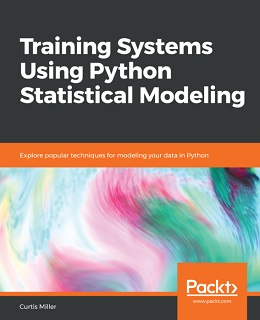# Training Systems using Python Statistical ModelingeBook Details:

eBook Description:

Training Systems using Python Statistical Modeling: Leverage the power of Python and statistical modeling techniques for building accurate predictive models

Python’s ease of use and multi-purpose nature has led it to become the choice of tool for many data scientists and machine learning developers today. Its rich libraries are widely used for data analysis, and more importantly, for building state-of-the-art predictive models. This book takes you through an exciting journey, of using these libraries to implement effective statistical models for predictive analytics.

You’ll start by diving into classical statistical analysis, where you will learn to compute descriptive statistics using pandas. You will look at supervised learning, where you will explore the principles of machine learning and train different machine learning models from scratch. You will also work with binary prediction models, such as data classification using k-nearest neighbors, decision trees, and random forests. This book also covers algorithms for regression analysis, such as ridge and lasso regression, and their implementation in Python. You will also learn how neural networks can be trained and deployed for more accurate predictions, and which Python libraries can be used to implement them.

By the end of this Training Systems using Python Statistical Modeling book, you will have all the knowledge you need to design, build, and deploy enterprise-grade statistical models for machine learning using Python and its rich ecosystem of libraries for predictive analytics.